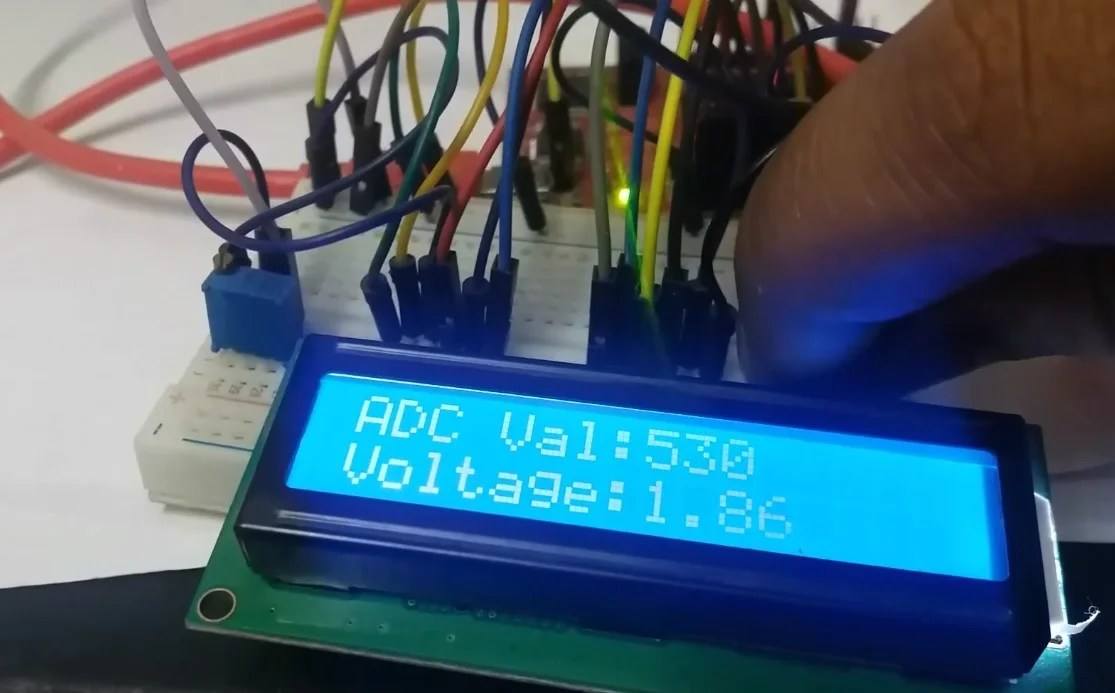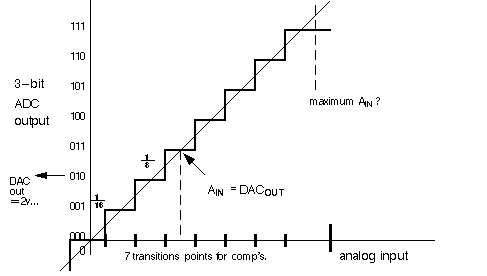# How to use ADC of MSP430 microcontroller

How to use ADC of MSP430 microcontroller, Analog to digital converter has many applications in the area of embedded systems and cyber physical systems. Analog to digital converters are used to measure analog signals. All microcontrollers  and microprocessors are digital devices. They only understand digital signals in binary form.  So for these devices to interact with analog world, we need to use ADCs which are acronym for analog to digital converts. For example you want to measure temperature and you are using LM35 temperature sensor. LM35 temperature sensor give analog voltage as a output. To measure temperature with the LM35 temperature sensor and MSP430 microcontroller, we need to find a way to interface analog output of temperature sensor with MSP430 microcontroller which only understands digital signals.Therefore MSP430 microcontroller has built in Analog to digital converter channels. These ADC channels used to convert analog digital into digital data. This digital data is equivalent of analog data.  So now you must have understand why we need ADC inside a microcontroller. If a microcontroller do not have built in ADC, we have to use external circuit which can convert analog signal into digital signal. But MSP430 series of microcontrollers have built more than one ADC channels. In this tutorial, we are use MSP430G2 launchpad which has on board MSP430G2553 microcontroller. MSP430G2553 microcontroller has eight analog channels on MSP430G2 launchPad. Its mean we can connect up to eight analog sensors with this microcontroller. In later part of this article, I will explain interfacing of potentiometer with MSP430 microcontroller and we will measuring voltage with one ADC channel of MSP430G2 board.

### Introduction to analog to digital converter or ADC

As I mentioned earlier ADC is a analog to digital converter which is used to convert analog signals of physical world into digital signals in the form of 1’s and 0’s.  Analog sensors like temperature sensor, hall effect sensor, pressure sensor, motion sensor used to measure physical world signals. But these physical world signals are converted into analog voltage by these sensor.  So to measure this analog voltage signals we need to use analog to digital converter.  So now lets see how analog to digital converter transform this analog signal into digital signal.

There are two important terms used for ADCs provided inside the integrated circuit of microcontrollers. One is adc channels and other one is resolution ADC.

### How to convert analog signal into digital signal with MSP430

Resolution of a microcontroller is define as minimum analog voltage a ADC can measure. For example if we have a 3 bit ADC and reference voltage is 5 volt.  The minimum voltage it can measure is

5 / 2^3 = 5 / 8 = 0.625 volt

So the minimum voltage a 3 bit analog channel can measure is 0.625 volt and any thing less than 0.625 volt microcontroller with 3 bit channel can not read.  But we we increase the number of bits of ADC, we can also increase its resolution.  Therefore high bits number ADCs are used to get higher accuracy.Picture above shows the each step conversion of 3 bit with reference voltage of 2 volt.

you may like to check other ADC related tutorials and projects:

### How to measure analog voltage with MSP430 microcontroller

So till now we have learnt that how ADCs converts analog voltage into digital value. So now lets see how to use Energia IDE to measure this voltage values.  We are using energia IDE in this series of tutorials. Energia IDE provides library for analog channel. So lets see the function and see how to use it.

• AnalogRead(Channel#) function : Analog read function is used to measure value of analog signal.
• As I mentioned earlier MSP430G2 launchPad has 8 analog channels A0, A1,A2, A3  to A7.
• We can use any channel to measure analog voltage.
• For example if you have used analog channel three or A3, you just need to mention AnalogRead(A3) inside this function to measure digital value from analog value and store the output of this function in integer variable. We will explain more about it in later part of this article.
• MSP430 launcpad has 8, 10 bit ADC channels and the reference voltage is 3.3 volt.
• So the resolution of MSP430 board analog channels is 3.3 / 1024 = 2.93mv
• So the minimum step is 2.93 mili volt and ADC counter increments by one for multiples of 2.93mv.
• AnalogReference function of Energia IDE can be used to change reference voltage less than 3.3 volt.

### How to interface potentiometer with MSP430G2 microcontroller

Now let see how to measure analog voltage with MSP430 microcontroller.  For demonstration purpose, we have connected a potentiometer with MSP430 and LCD to display digital value and analog voltage.  I recommend  you to check this LCD interfacing with MSP430G2 launchPad tutorial if you don’t know about LCD interfacing.For more explanation and about the working of code, watch this complete lecture.

• In this lecture first, we will learn about the interfacing of potentiomter with MSP430.
• After that you will see an example of how to measure voltage across variable resistor and how to display value of voltage and ADC value on LCD.

### 1 thought on “How to use ADC of MSP430 microcontroller”

1. My name is Hariz Azmi and a student. Currently do this project. Can you help me do this project? Really need your help. I try do my best to learn. Hope can contact you.
Thank you.